# Binary Lesson 7 Review of Binary and Hexadecimal

• Slides: 20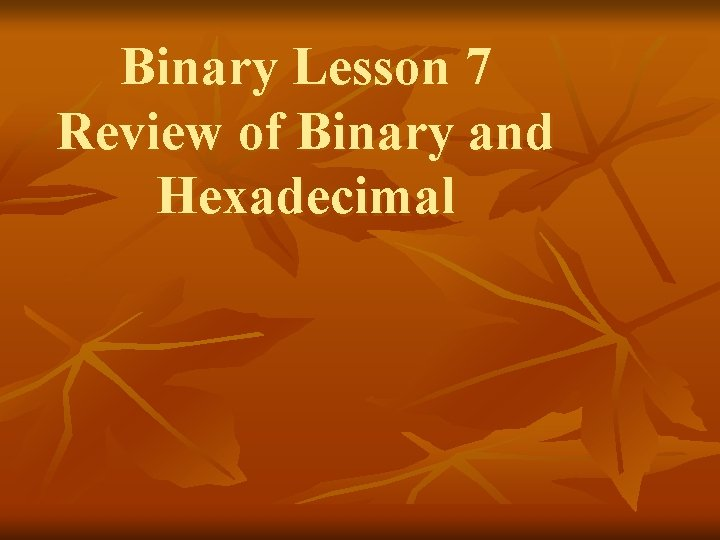Binary Lesson 7 Review of Binary and Hexadecimal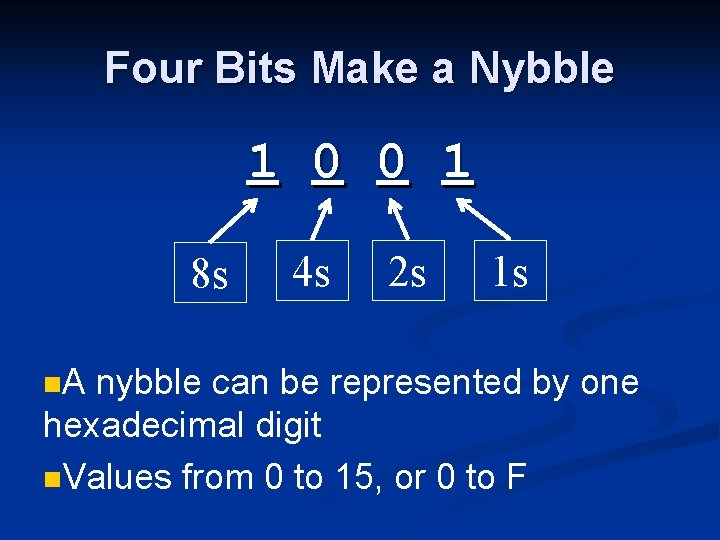Four Bits Make a Nybble 1 0 0 1 8 s n. A 4 s 2 s 1 s nybble can be represented by one hexadecimal digit n. Values from 0 to 15, or 0 to F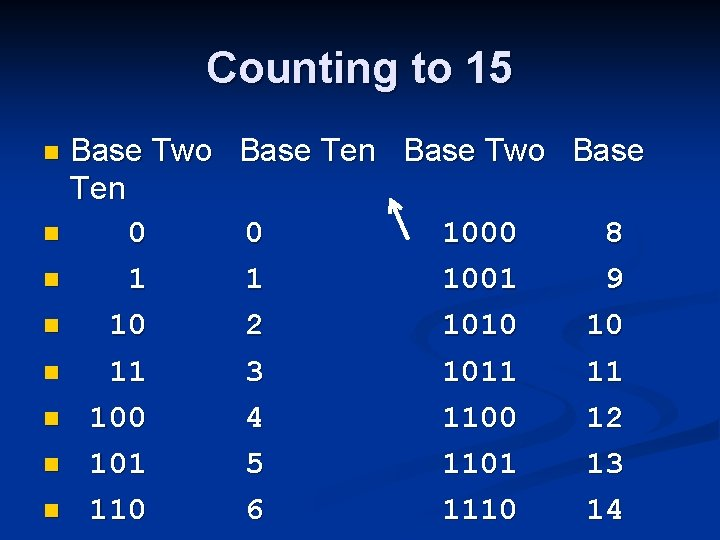Counting to 15 Base Two Ten n 0 n 10 n 11 n 100 n 101 n 110 n Base Ten Base Two Base 0 1 2 3 4 5 6 1000 1001 1010 1011 1100 1101 1110 8 9 10 11 12 13 14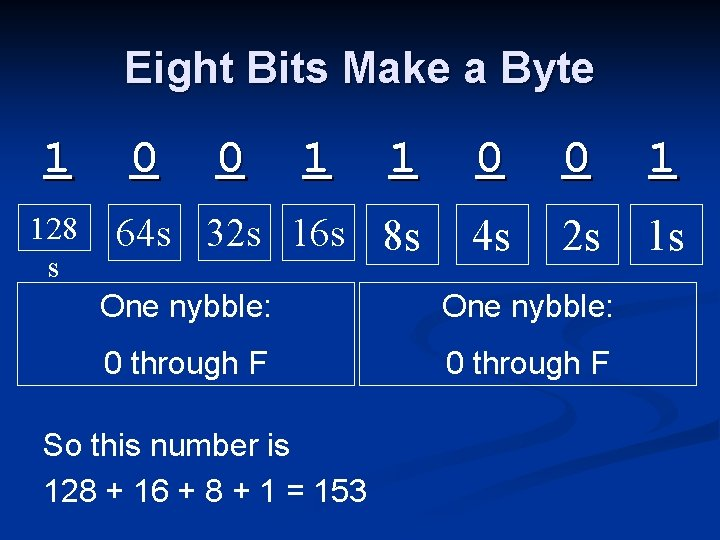Eight Bits Make a Byte 1 128 s 0 0 1 1 0 0 1 64 s 32 s 16 s 8 s 4 s 2 s 1 s One nybble: 0 through F So this number is 128 + 16 + 8 + 1 = 153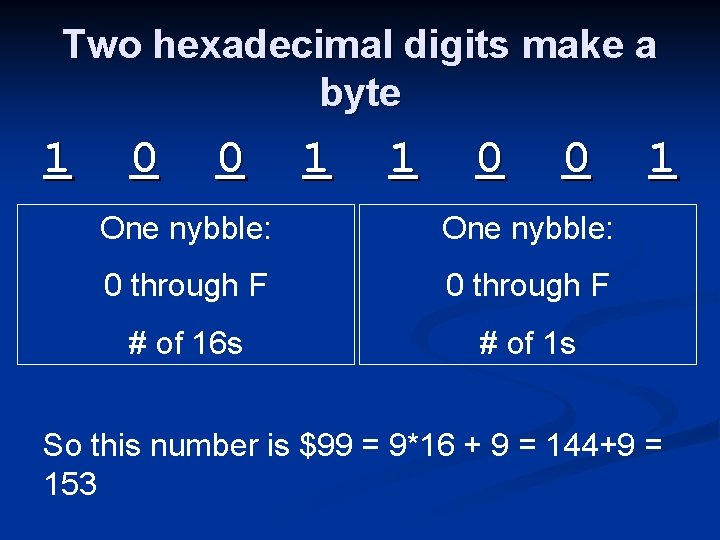Two hexadecimal digits make a byte 1 0 0 1 1 0 0 One nybble: 0 through F # of 16 s # of 1 s 1 So this number is \$99 = 9*16 + 9 = 144+9 = 153Review of Binary and Hexadecimal i. Clicker Questions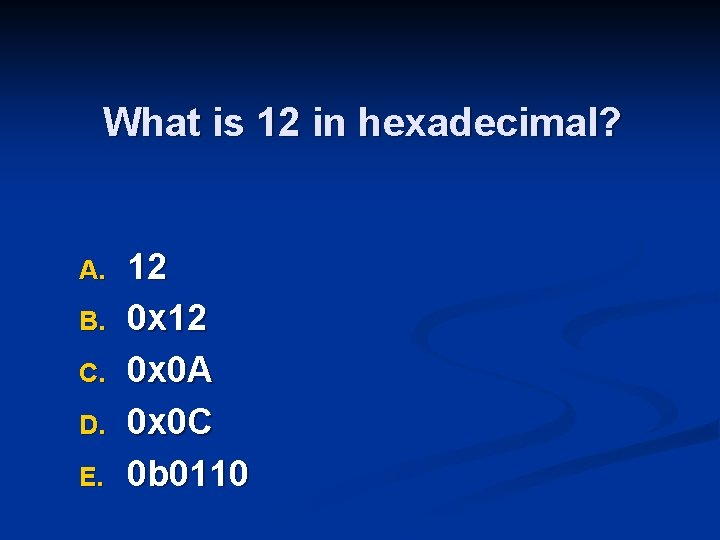What is 12 in hexadecimal? A. B. C. D. E. 12 0 x 0 A 0 x 0 C 0 b 0110What is 0 b 1111 in decimal? A. B. C. D. E. 11 13 15 0 x 0 F Something else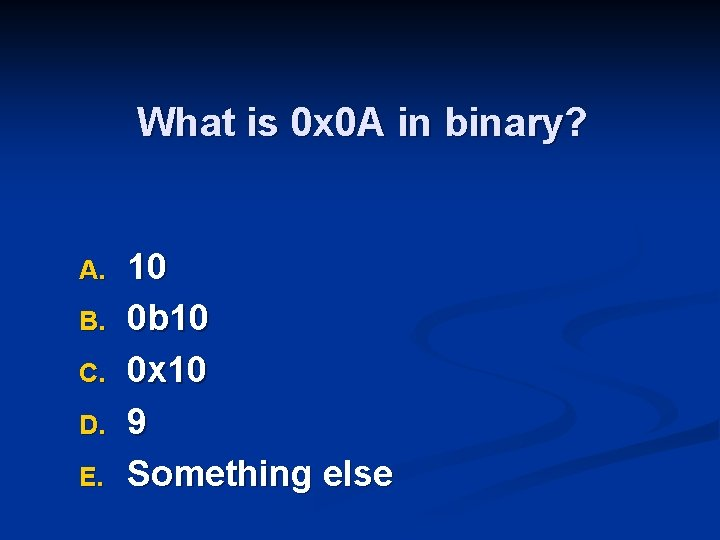What is 0 x 0 A in binary? A. B. C. D. E. 10 0 b 10 0 x 10 9 Something else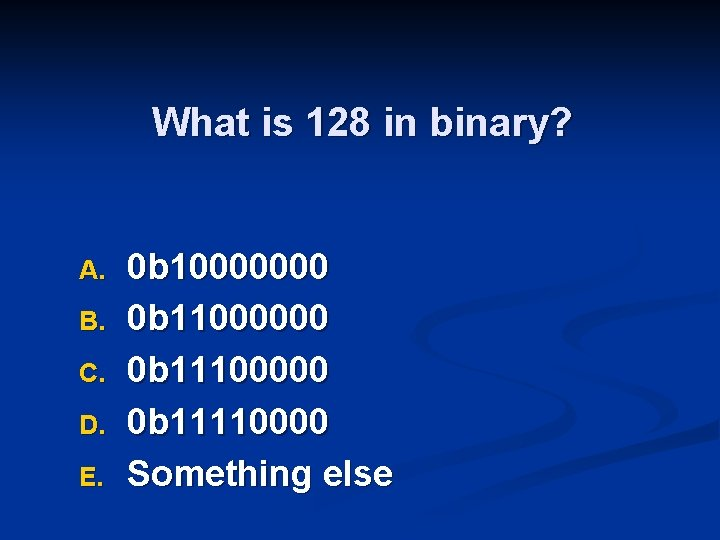What is 128 in binary? A. B. C. D. E. 0 b 10000000 0 b 11100000 0 b 11110000 Something else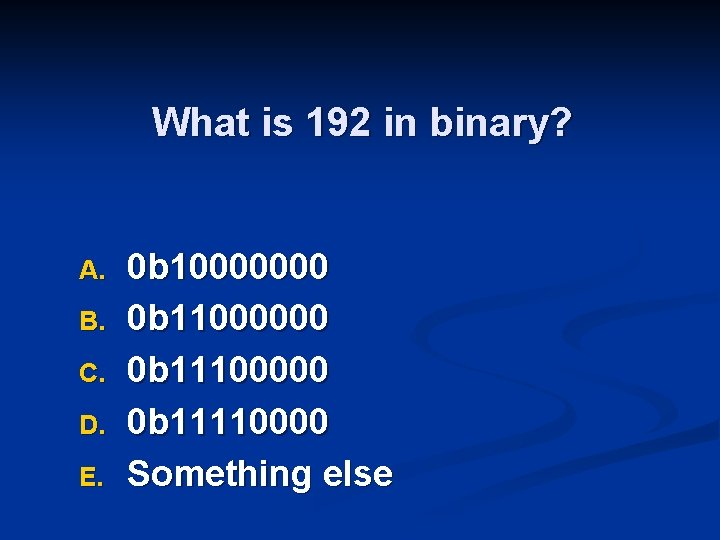What is 192 in binary? A. B. C. D. E. 0 b 10000000 0 b 11100000 0 b 11110000 Something elseWhat is 160 in hex? A. B. C. D. E. 0 x 0 A 0 x 10 0 x. AA Something elseWhat is 168 in hex? A. B. C. D. E. 0 x 0 A 0 x. A 2 0 x. A 8 0 x. AA Something else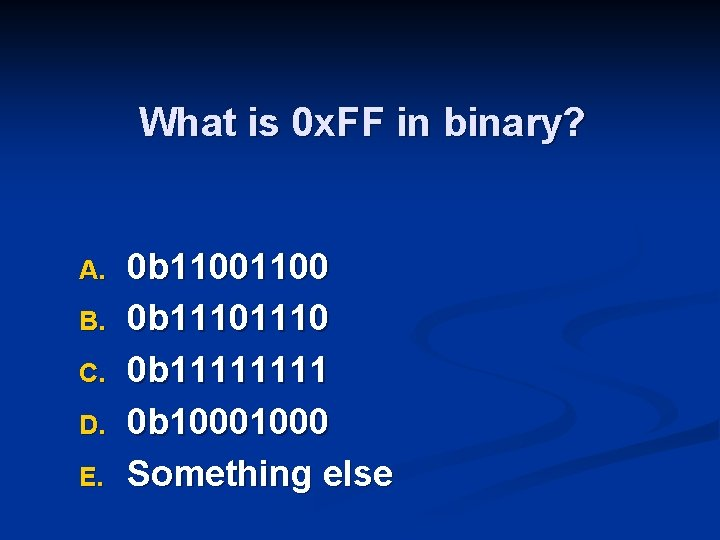What is 0 x. FF in binary? A. B. C. D. E. 0 b 1100 0 b 1110 0 b 1111 0 b 1000 Something else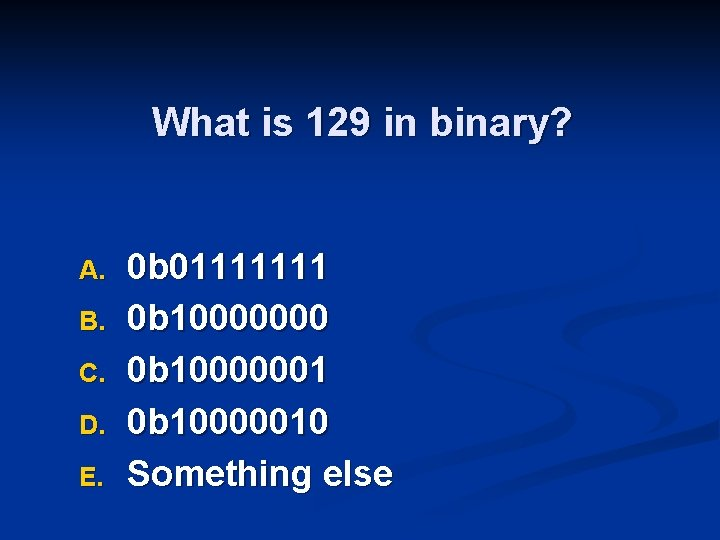What is 129 in binary? A. B. C. D. E. 0 b 01111111 0 b 10000000 0 b 10000001 0 b 10000010 Something else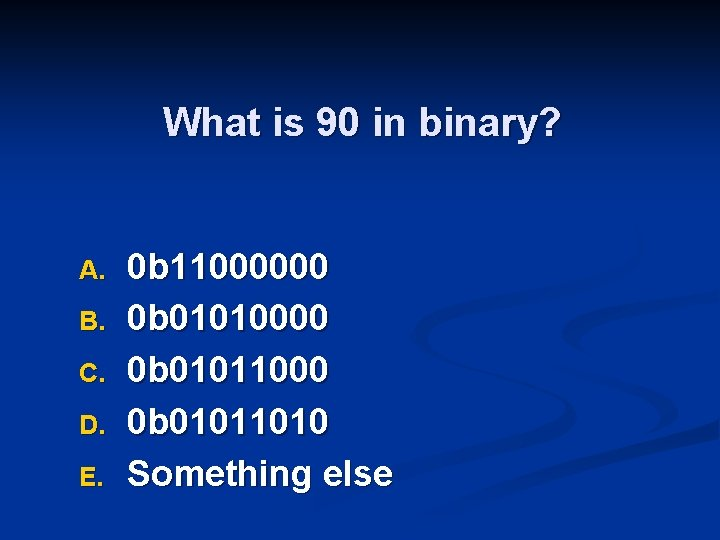What is 90 in binary? A. B. C. D. E. 0 b 11000000 0 b 01011000 0 b 01011010 Something else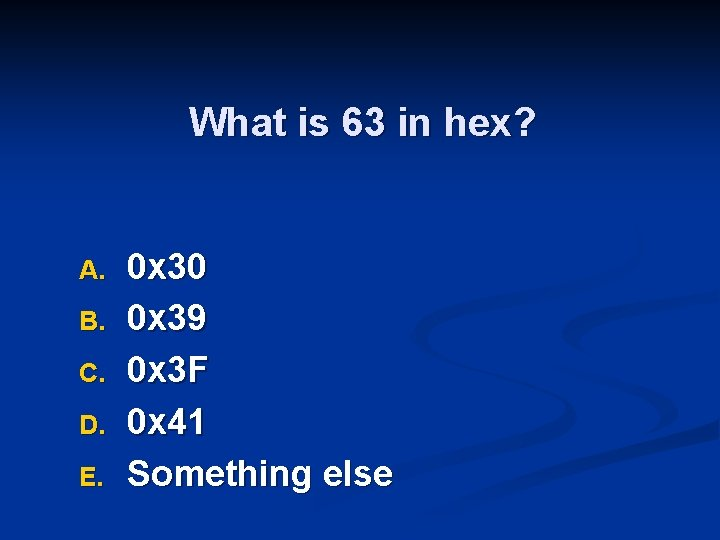What is 63 in hex? A. B. C. D. E. 0 x 30 0 x 39 0 x 3 F 0 x 41 Something else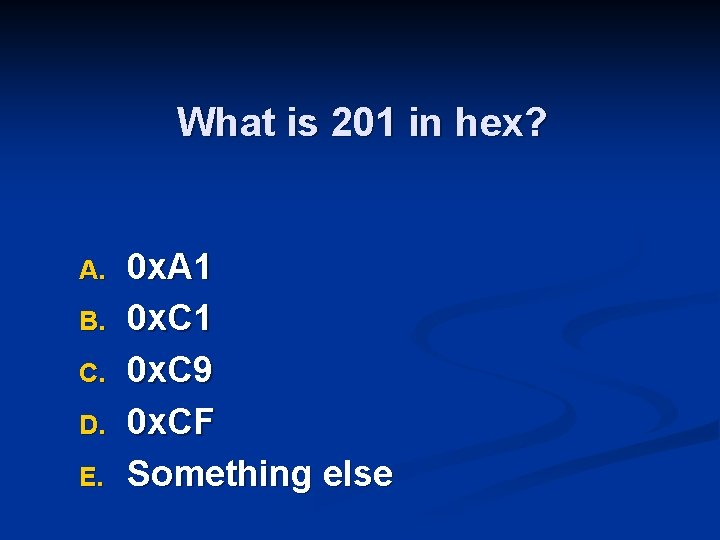What is 201 in hex? A. B. C. D. E. 0 x. A 1 0 x. C 9 0 x. CF Something elseWhat is 0 x. F 1 in binary? A. B. C. D. E. 0 b 1111 0 b 1110 0 b 11110011 0 b 11110001 Something else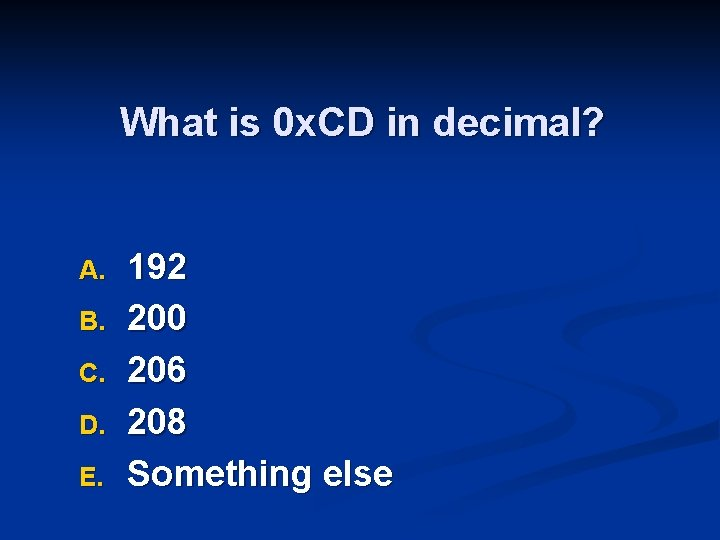What is 0 x. CD in decimal? A. B. C. D. E. 192 200 206 208 Something else